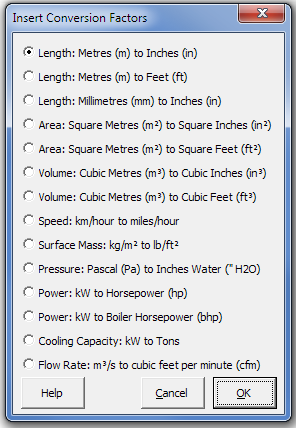### Strutt Help

Conversion Factors    1/1, 1/3

Strutt|Fundamentals|Conversion Factors inserts a conversion factor between US customary and SI units into the current row of the worksheet. The current available conversion factors are:Conversion factors are 'multiplication' factors - i.e. the value in the first unit is multiplied by the conversion factor to obtain the value in the second unit. E.g. the conversion factor between metres (m) to inches (in) is 39.4; 1 m is equivalent to 39.4 in.